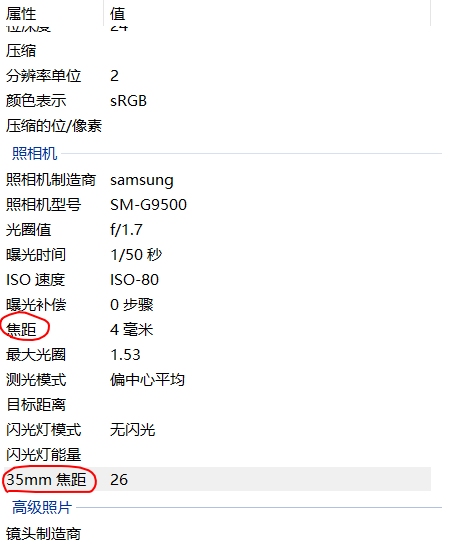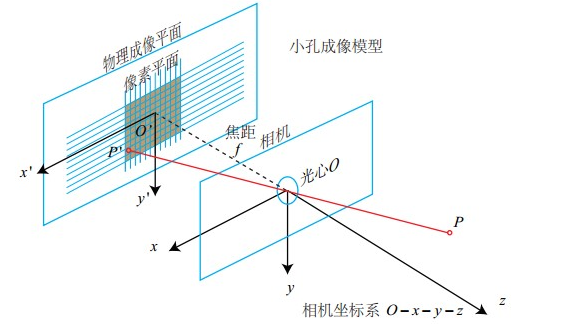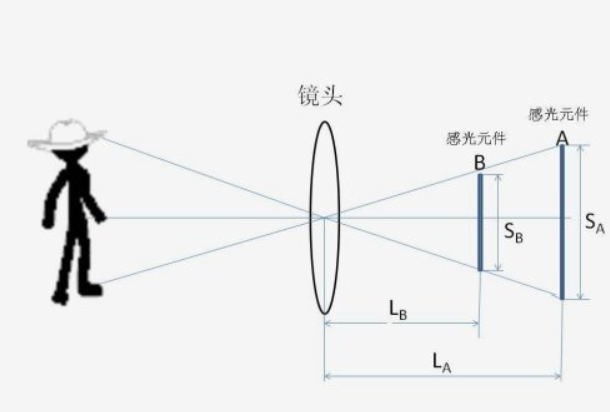# SFM之通过exif获取初始焦距

在SFM的过程中，由于相机是没有进行标定的，因此我们通常通过图片的exif信息（一般相机拍出来的原始图像都会有这个信息）来读取相机的焦距信息。本文不讲述如何读取exif信息，只说明如何通过已得到的exif信息计算我们小孔成像模型的初始焦距。### 1.通过焦距$f_{FocalLength}$这是相机的小孔成像模型，上图中的物理成像平面就是真实相机中镜头后的感光元件，由CCD或CMOS组成，上图中的$f$就是exif文件中的焦距$f_{FocalLength}$，我们不妨设这块感光元件的宽为$w$，高为$h$，单位为毫米。同时设水平方向$w_p$个像素，垂直方向$h_p$个像素。由于像素通常是正方形，所以有：
$\displaystyle\frac{w}{w_p}=\frac{h}{h_p}$

$X'=f\displaystyle\frac{X}{Z}$

$u=\displaystyle\frac{w_p}{w}X'=\displaystyle\frac{w_p}{w}f\displaystyle\frac{X}{Z}\\ v=\displaystyle\frac{h_p}{h}Y'=\displaystyle\frac{h_p}{h}f\displaystyle\frac{Y}{Z}=\displaystyle\frac{w_p}{w}f\displaystyle\frac{Y}{Z}$

### 2.通过等效35mm焦距$f_{35mm}$

那么如果我们的备用文件里没有相应型号怎么办呢？这时就可以通过等效35mm焦距$f_{35mm}$来近似求取$f_x$了。下面介绍一下什么是等效35mm焦距：$\displaystyle\frac{f_A}{S_A}=\displaystyle\frac{f_B}{S_B}$

©️2019 CSDN 皮肤主题: 大白 设计师: CSDN官方博客